# Maharashtra Board 8th Class Maths Practice Set 8.1 Solutions Chapter 8 Quadrilateral: Constructions and Types

Balbharti Maharashtra State Board Class 8 Maths Solutions covers the Practice Set 8.1 8th Std Maths Answers Solutions Chapter 8 Quadrilateral: Constructions and Types.

## Practice Set 8.1 8th Std Maths Answers Chapter 8 Quadrilateral: Constructions and Types

Construct the following quadrilaterals of given measures.

Question 1.
In ∆MORE, l(MO) = 5.8 cm, l(OR) = 4.4 cm, m∠M = 58°, m∠O = 105°, m∠R = 90°.
Solution:Question 2.
Construct ∆DEFG such that l(DE) = 4.5 cm, l(EF) = 6.5 cm, l(DG) = 5.5 cm, l(DF) = 7.2 cm, l(EG) = 7.8 cm.
Solution:Question 3.
In ∆ABCD, l(AB) = 6.4 cm, l(BC) = 4.8 cm, m∠A = 70°, m∠B = 50°, m∠C = 140°.
Solution: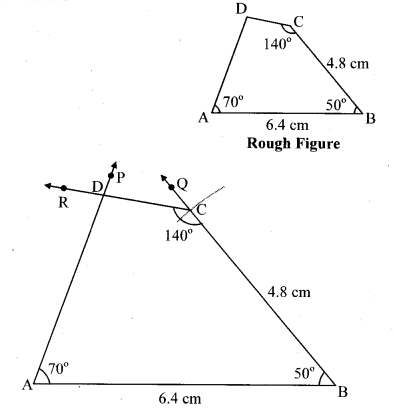Question 4.
Construct ₹LMNO such that
l(LM) = l(LO) = 6 cm,
l(ON) = l(NM) = 4.5 cm, l(OM) = 7.5 cm.
Solution:Maharashtra Board Class 8 Maths Chapter 8 Quadrilateral: Constructions and Types Practice Set 8.1 Intext Questions and Activities

Question 1.
Construction of a triangle:
Construct the triangles with given measures. (Textbook pg. no. 41)
i. ∆ABC: l(AB) = 5 cm, l(BC) = 5.5, l(AC) = 6 cm.
Solution:Steps of construction:
Step 1 : As shown in the rough figure, draw seg BC of length 5.5 cm as the base.
Step 2 : By taking a distance of 5 cm on the compass and placing the metal tip of the compass on point B, draw an arc on one side of BC.
Step 3 : By taking a distance 6 cm on the compass and placing the metal tip of the t compass on point C and draw an arc ’ such that it intersects the previous arc. Name the point as A.
Step 4 : Draw segments AB and AC to get the triangle. ∆ABC is the required triangle.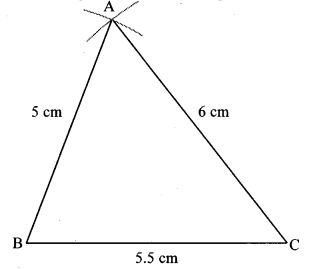ii. ∆DEF: m∠D = 35°, m∠F = 100°, l(DF) = 4.8 cm.
Solution: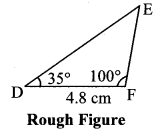Steps of construction:
Step 1 : As shown in the rough figure, draw seg DF of length 4.8 cm as the base.
Step 2 : Placing the centre of the protractor at point D, mark point P such that m∠PDF = 35°.
Step 3 : Placing the centre of the protractor at point F, mark point Q such that m∠QFD = 100°.
Step 4 : Draw ray DP and ray FQ. Name their point of intersection as E.
∆DEF is required triangle.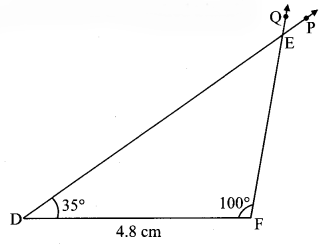iii. ∆MNP: l(MP) = 6.2 cm, l(NP) = 4.5 cm, m∠P = 75°.
Solution:Steps of construction:
Step 1 : As shown in the rough figure, draw seg PN of length 4.5 cm as the base.
Step 2 : Placing the centre of the protractor at point P, mark point Q such that m∠QPN = 75°.
Step 3 : By taking a distance of 6.2 cm on the compass and placing the metal tip at point P, draw an arc on ray PQ. Name the point as M.
Step 4 : Draw seg MN to get the triangle. ∆MNP is the required triangle.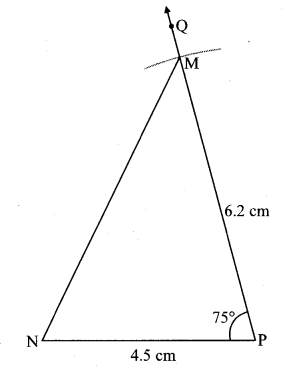iv. ∆XYZ: m∠Y = 90°, l(XY) = 4.2 cm, l(XZ) = 7 cm.
Solution:Steps of construction:
Step 1 : As shown in the rough figure, draw seg XY of 4.2 cm as the base.
Step 2 : Placing the centre of the protractor at point Y, mark point Q such that m∠QYX = 90°.
Step 3 : By taking a distance of 7 cm on the compass and placing the metal tip on point X, draw an arc on ray YQ. Name the point as Z.
Step 4 : Draw seg XZ to get the triangle. ∆XYZ is the required triangle.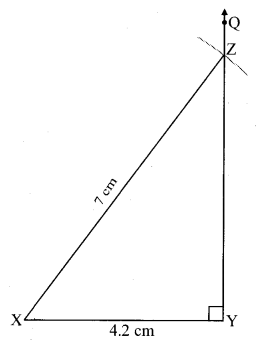Scroll to Top